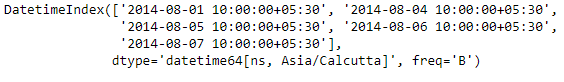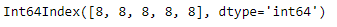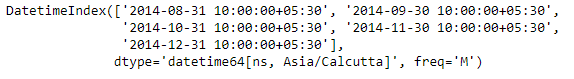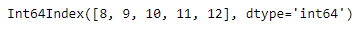Python | Pandas DatetimeIndex.month

• Last Updated : 24 Dec, 2018

Python is a great language for doing data analysis, primarily because of the fantastic ecosystem of data-centric python packages. Pandas is one of those packages and makes importing and analyzing data much easier.

Pandas DatetimeIndex.month attribute outputs an Index object containing numeric values corresponding to each entry in the DatetimeIndex object. It outputs the month as January=1, December=12 and corresponding numeric values for each month in between.

Syntax: DatetimeIndex.month

Return: Index containing months.

Example #1: Use DatetimeIndex.month attribute to find the months present in the DatetimeIndex.

 # importing pandas as pdimport pandas as pd  # Create the DatetimeIndex# Here the 'B' represents Business day frequencydidx = pd.DatetimeIndex(start ='2014-08-01 10:00', freq ='B',                            periods = 5, tz ='Asia/Calcutta')  # Print the DatetimeIndexprint(didx)

Output :Now we want to find all the month values present in the DatetimeIndex object.

 # find all the months in the objectdidx.month

Output :As we can see in the output, the function has returned an Index object containing the numeric value corresponding to months of each entry in the DatetimeIndex object.

Example #2: Use DatetimeIndex.month attribute to find the months present in the DatetimeIndex.

 # importing pandas as pdimport pandas as pd  # Create the DatetimeIndex# Here the 'M' represents Month end frequencydidx = pd.DatetimeIndex(start ='2014-08-01 10:00', freq ='M',                           periods = 5, tz ='Asia/Calcutta')  # Print the DatetimeIndexprint(didx)

Output :Now we want to find all the month values present in the DatetimeIndex object.

 # find all the months in the objectdidx.month

Output :As we can see in the output, the function has returned an Index object containing the numeric value corresponding to months of each entry in the DatetimeIndex object.

Attention geek! Strengthen your foundations with the Python Programming Foundation Course and learn the basics.

To begin with, your interview preparations Enhance your Data Structures concepts with the Python DS Course. And to begin with your Machine Learning Journey, join the Machine Learning - Basic Level Course

My Personal Notes arrow_drop_up